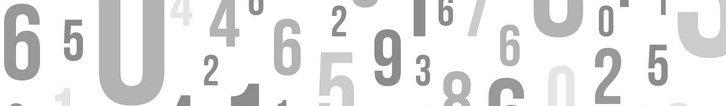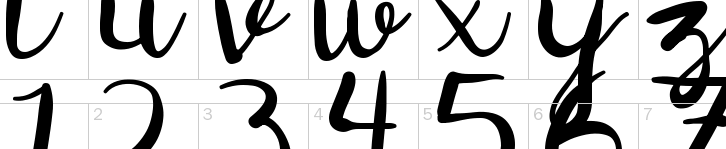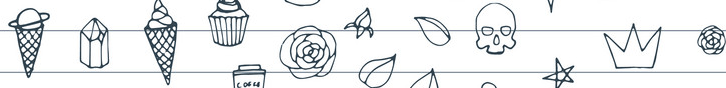C# Tutorial

# Random strings, Alphanumeric Strings and Numbers

The random string creates a series of numbers, letters and alphanumeric strings that have no pattern. These can be helpful for creating security codes, passwords etc. The generation of this type of random string can be a common or typical task in C# programming . Some forms of randomness concern hash or search algorithms.

## C# Random Class

C# Random class returns a pseudo-random number generator, which is an algorithm that produces a sequence of numbers that meet certain statistical requirements for randomness. The Next() method in Random class returns a non-negative random number . We can limit the generation of Random number by giving a specified range to Next() method.

random.Next(0,10);

The above code limit to generate the Random number only within the specified range from 0 to 10. Using this C# Random Class one can generate a different set of numbers/characters etc. There are many different ways of generating random strings in the C# language. The following C# random string generator programs creates a bunch of examples that generates random numbers, strings and random alphanumeric strings random alphanumeric strings based on the configuration parameters that you specified.example 1

## C# Random String

A simple program to generate random string of length 16 characters using C# Random Class .

Random random = new Random(); int length = 16; var rString = ""; for (var i = 0; i < length; i++) { rString += ((char)(random.Next(1, 26) + 64)).ToString().ToLower(); } MessageBox.Show(rString);
Full Source
using System; using System.Windows.Forms; namespace WindowsFormsApplication { public partial class Form1 : Form { public Form1() { InitializeComponent(); } private void button1_Click_1(object sender, EventArgs e) { Random RNG = new Random(); int length = 16; var rString = ""; for (var i = 0; i < length; i++) { rString += ((char)(RNG.Next(1, 26) + 64)).ToString().ToLower(); } MessageBox.Show(rString); } } }
example 2

## C# Random Alphanumeric String

The following program generate random alphanumeric strings using C# Random Class.

const string src = "abcdefghijklmnopqrstuvwxyz0123456789"; int length = 16; var sb = new StringBuilder(); Random RNG = new Random(); for (var i = 0; i < length; i++) { var c = src[RNG.Next(0, src.Length)]; sb.Append(c); } MessageBox.Show(sb.ToString());
Full Source
using System; using System.Windows.Forms; using System.Text; namespace WindowsFormsApplication { public partial class Form1 : Form { public Form1() { InitializeComponent(); } private void button1_Click_1(object sender, EventArgs e) { const string src = "abcdefghijklmnopqrstuvwxyz0123456789"; int length = 16; var sb = new StringBuilder(); Random RNG = new Random(); for (var i = 0; i < length; i++) { var c = src[RNG.Next(0, src.Length)]; sb.Append(c); } MessageBox.Show(sb.ToString()); } } }
example 3

## Using Path.GetRandomFileName

The C# Path.GetRandomFileName method returns a cryptographically strong, random string that can be used as either a folder name or a file name. The filename does not conflict with other files. Here you can use this method to generate random strings .

string rString = Path.GetRandomFileName(); rString = rString.Replace(".", ""); // Remove period. MessageBox.Show(rString);
Full Source
using System; using System.Windows.Forms; using System.IO; namespace WindowsFormsApplication { public partial class Form1 : Form { public Form1() { InitializeComponent(); } private void button1_Click_1(object sender, EventArgs e) { string rString = Path.GetRandomFileName(); rString = rString.Replace(".", ""); // Remove period. MessageBox.Show(rString); } } }
example 4

## Generating Random Numbers and Strings Using GUID

A GUID (Global Unique Identifier) is a 128-bit integer (16 bytes) that can be used across all computers and networks wherever a unique identifier is required. Such an identifier has a very low probability of being duplicated and it cannot be easily guessed.

Guid guid = Guid.NewGuid(); string rString = Convert.ToBase64String(guid.ToByteArray()); rString = rString.Replace("=", ""); //remove '=' MessageBox.Show(rString);
Full Source
using System; using System.Windows.Forms; namespace WindowsFormsApplication { public partial class Form1 : Form { public Form1() { InitializeComponent(); } private void button1_Click_1(object sender, EventArgs e) { Guid guid = Guid.NewGuid(); string rString = Convert.ToBase64String(guid.ToByteArray()); rString = rString.Replace("=", ""); //remove '=' MessageBox.Show(rString); } } }example 5

## Generating Random Strings using LinQ

Enumerable.Range method on the Enumerable type to get a range of numbers. The immediate return value is an object that stores all the information that is required to perform the action. By using Enumerable.Range method, you can generate random strings and alphanumeric strings .

Random RNG = new Random(); const string range = "abcdefghijklmnopqrstuvwxyz0123456789"; var chars = Enumerable.Range(0, 20).Select(x => range[RNG.Next(0, range.Length)]); MessageBox.Show(new string(chars.ToArray()));
Full Source
using System; using System.Windows.Forms; using System.Linq; namespace WindowsFormsApplication { public partial class Form1 : Form { public Form1() { InitializeComponent(); } private void button1_Click_1(object sender, EventArgs e) { Random RNG = new Random(); const string range = "abcdefghijklmnopqrstuvwxyz0123456789"; var chars = Enumerable.Range(0, 20).Select(x => range[RNG.Next(0, range.Length)]); MessageBox.Show(new string(chars.ToArray())); } } }
example 6

## Generate random (numbers+Alphanumeric) using LinQ

Enumerable.Range method on the Enumerable type to get a range of numbers. By using Enumerable.Range method, you can generate random string including numbers and special characters.

var r = new Random(); string rString = new String(Enumerable.Range(0, 24).Select(n => (Char)(r.Next(32, 127))).ToArray()); MessageBox.Show(rString);
Full Source
using System; using System.Windows.Forms; using System.Linq; namespace WindowsFormsApplication { public partial class Form1 : Form { public Form1() { InitializeComponent(); } private void button1_Click_1(object sender, EventArgs e) { var RNG = new Random(); var rString = Enumerable.Range(0, 24).Select(n => (Char)(RNG.Next(32, 127))); MessageBox.Show(new string(rString.ToArray())); } } }
example 7

## Using RNGCryptoServiceProvider Class

RNGCryptoServiceProvider generates high-quality random numbers. Implements a RNG ( cryptographic Random Number Generator ) using the implementation provided by the cryptographic service provider (CSP). The most useful method on RNGCryptoServiceProvider is the GetBytes method because this type implements Dispose, you can enclose it in a using-statement.

var rBytes = new byte; using (var crypto = new RNGCryptoServiceProvider()) crypto.GetBytes(rBytes); var base64 = Convert.ToBase64String(rBytes); MessageBox.Show(base64); // Generate Alphanumeric string: var result = Regex.Replace(base64, "[A-Za-z0-9]", ""); MessageBox.Show(result);
Full Source
using System; using System.Windows.Forms; using System.Text.RegularExpressions; using System.Security.Cryptography; namespace WindowsFormsApplication { public partial class Form1 : Form { public Form1() { InitializeComponent(); } private void button1_Click_1(object sender, EventArgs e) { var rBytes = new byte; using (var crypto = new RNGCryptoServiceProvider()) crypto.GetBytes(rBytes); var base64 = Convert.ToBase64String(rBytes); MessageBox.Show(base64); // Generate Alphanumeric string: var result = Regex.Replace(base64, "[A-Za-z0-9]", ""); MessageBox.Show(result); } } }example 8

## Generate Random Number in C#

Finally just generate C# Random Numbers only within a specified range.

using System; using System.Windows.Forms; namespace WindowsFormsApplication { public partial class Form1 : Form { public Form1() { InitializeComponent(); } private void button1_Click_1(object sender, EventArgs e) { Random random = new Random(); for (int i = 0; i < 5; i++) { MessageBox.Show(Convert.ToString(random.Next(10, 20))); } } } }